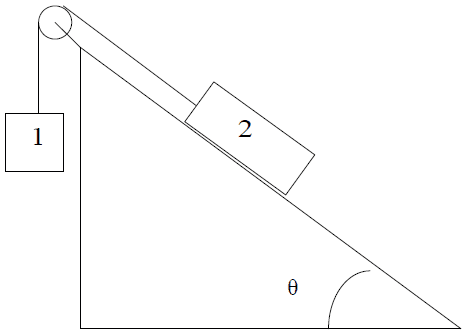# Tension of a string with a pulley

asdf12321asdf

## Homework Statement

Two masses are connected by a string over a pulley as shown below. The incline is
frictionless and makes a 50 degree angle from the horizontal. If m1 = 3.00 kg and m2 = 8.00 kg, find the tension in the string.F = ma

## The Attempt at a Solution

I thought the tension in the string would be the same as the weight of m1, which is 29.4N, but that is not the correct answer. I am not sure what to try from here.

Thaakisfox
m1 accelerates, so you need to take that into consideration.
First write up the equations of motion of the two blocks.
i.e. m1*a=...
and m2*a=...

asdf12321asdf
I got that that a equals 2.79m/s², so:

m1*a = 3 * 2.79 = 8.37N
m2*a = 8 * 2.79 = 22.32N

Is that what you mean? Is that right?

Thaakisfox
Yes, and now you can express the tension of the string. m1*a=m1*g-T

asdf12321asdf
That got me the correct answer! Thanks so much for your help!

Last edited: